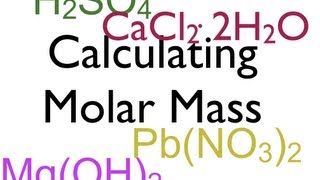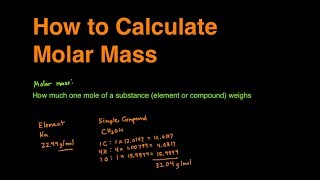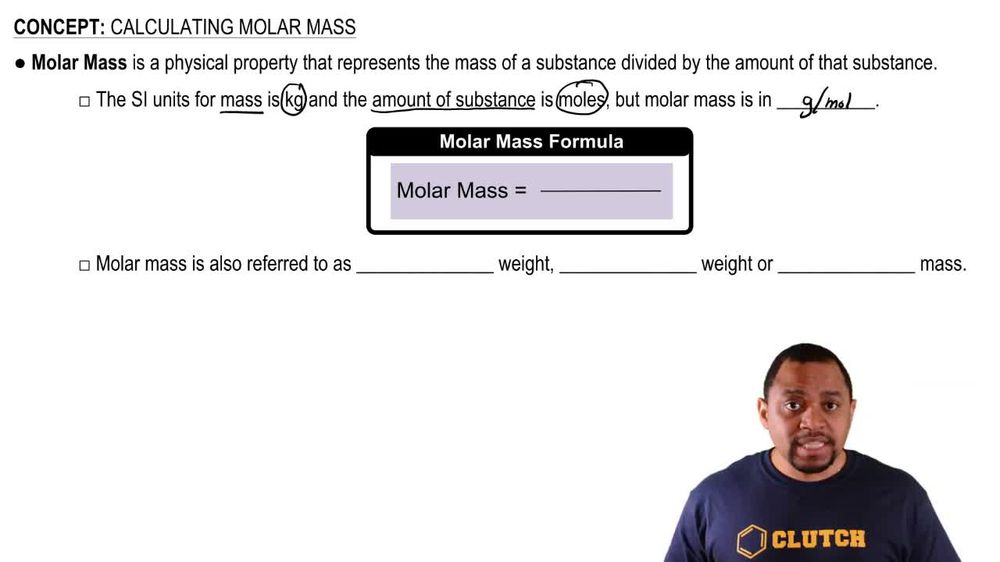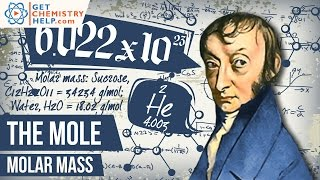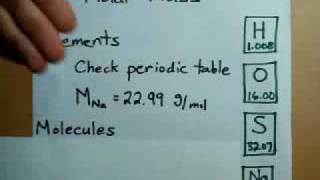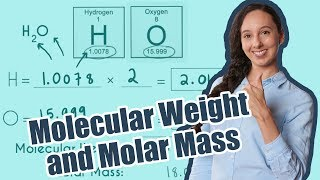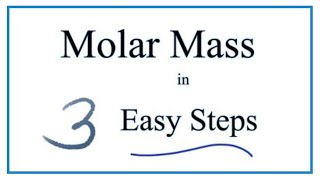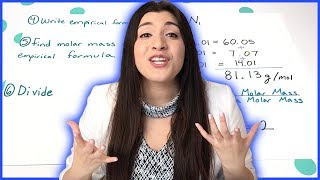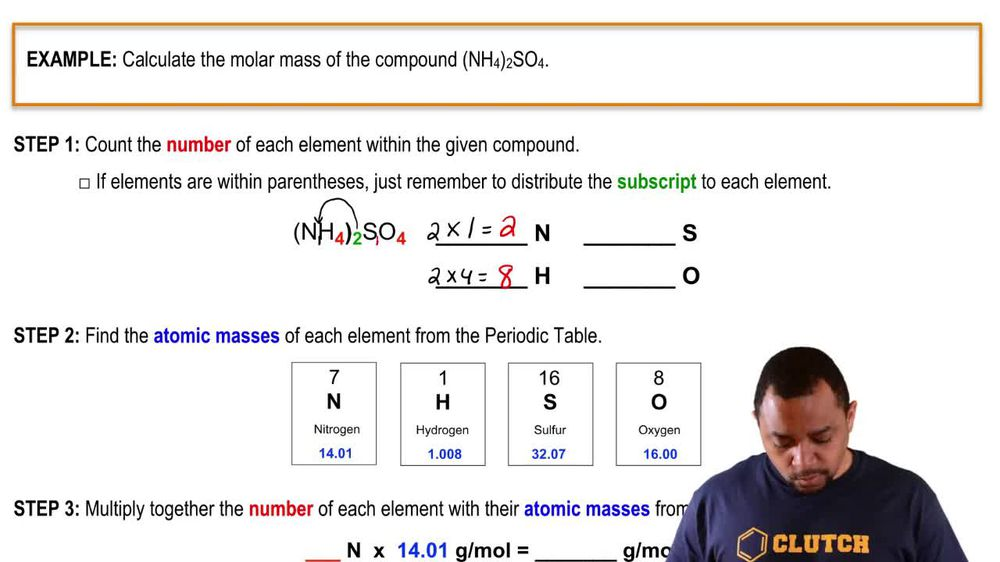Start typing, then use the up and down arrows to select an option from the list.
1. 2. Atoms & Elements2. Calculating Molar Mass
Problem

# What is the molecular weight of chloroform if 0.0275 mol weighs 3.28 g?

Relevant Solution1m
Play a video:
Hey everyone in this example, we're told that if 1.85 times 10 to the third power moles 10 to the negative third power moles of a hormone ways 100.5 81 g, calculate the molar mass of the hormone. We want to recall that molar mass, which is sometimes represented as a capital M is going to be equal to our mass. That is given divided by moles which are in units of grams per mole. So what we're going to find for our molar mass of the hormone Is that it's going to be equal to the given mass in the prompt as 0.581 g. And we're going to divide this by our moles of our hormone given in the prompt as 1.85 times 10 to the negative third power moles. And as you can see, we have the units g per mole, which is what we want. And this is going to give us a value equal to g per mole as our molar mass of our hormone. To complete this example as our final answer. So I hope that everything I reviewed was clear. If you have any questions, please leave them down below and I will see everyone in the next practice video